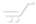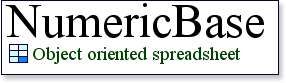# Instantiated tables

Instantiated tables
Instantiated tables let you use an existing table as function. You do this by instantiating (duplicating) an existing table, and then overriding some of the table cells to use the existing table functionally under different parameters.

The instantiated table syntax.
To use instantiated table, you need to use the following syntax, which is an expansion of the standard function call syntax. It has the following elements :
1. Table name
2. The left parentheses sign (
3. List of named arguments separated by commas. Each named arguments consist of:
• Name that corresponds to a column name of the table.
• The assignment operator :=
• formula.
4. the right parentheses sign )
Example
For example, if your solution has the temp_convert table of the following screenshot, (based on example from the circular dependency page), you can supply the Celsius degrees and get the Fahrenheit back:
temp_convert(Celsius:=0).Fahrenheit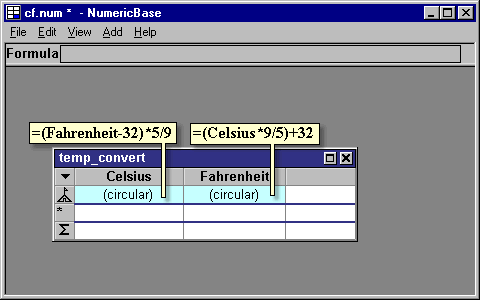Screenshot: the temp_convert table used in example

Evaluation of instantiated table.
When MumericBase evaluates the formula
temp_convert(Celsius:=0).Fahrenheit
, it instantiates a temp_convert table, and set the value of the Celsius column at the base row to be 0. It then uses the dot access operator to extract the Fahrenheit value from this instantiated table. Note that this instantiated table is a duplicate of temp_convert, and the original one stays intact.

The following screenshot shows this formula on row 1 of Table1. It also show an alternative: first create the table, using the formula
=temp_convert(Celsius:=0)
, and then extract the value for that table, using the formula =prev.Fahrenheit. The table icon on row 2, indicates a reference the instantiated table.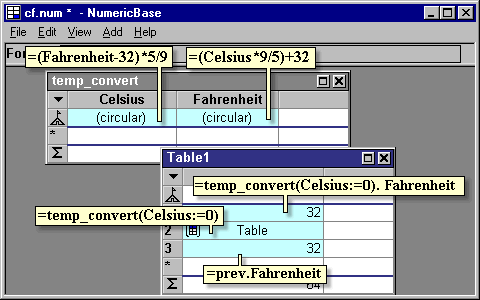Screenshot: using the temp_convert table

Viewing the instantiated table
You can see the instantiated table in more details by clicking it's icon, as shown in the following animated screenshot. Note the different background color of the Celsius column. This color is to indicate that the value was overridden using a instantiated table parameter. This different background color is expansion of the color coding feature.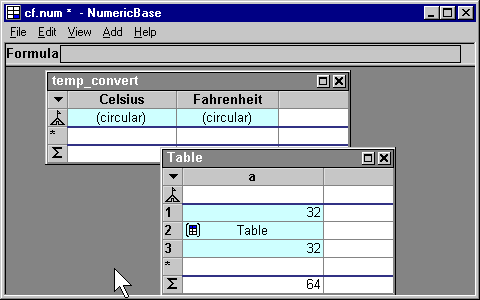Screenshot: viewing the instantiated table

Another example
For a more elaborate example, see the saving account scenarios example - note the formula Table2(intrest:=interest) at the tables column.